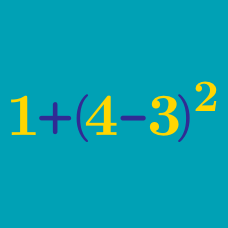Basic Mathematics

# Basic Arithmetic: Level 1 Challenges

$\Large \text{Compute } \ 3 + 0 \times 5 + 5 - 9.$

$2+2\div2=?$

$\LARGE (\color{#D61F06}2^{\color{#20A900}4})^{\color{#3D99F6}8} \div ({\color{#20A900}4}^{\color{#3D99F6}8})^{\color{#D61F06}2} = \ \color{grey}?$

When I turned 2 years old, my brother was just half of my age.

Today I turned 19 years old. How old is my brother?

$\large \text{ Is } 2^{999} \text{ bigger than } 999^2 ?$

×